# 选择排序介绍和稳定性说明

• 如果相同，则是稳定的排序方法。
• 如果不相同，则是不稳定的排序方法

## 一、第一趟排序



int[] arrays = {2, 3, 1, 4, 3, 5, 1, 6, 1, 2, 3, 7, 2, 3};

//假定max是最大的
int max = 0;

for (int i = 0; i < arrays.length; i++) {
if (arrays[i] > max) {
max = arrays[i];
}
}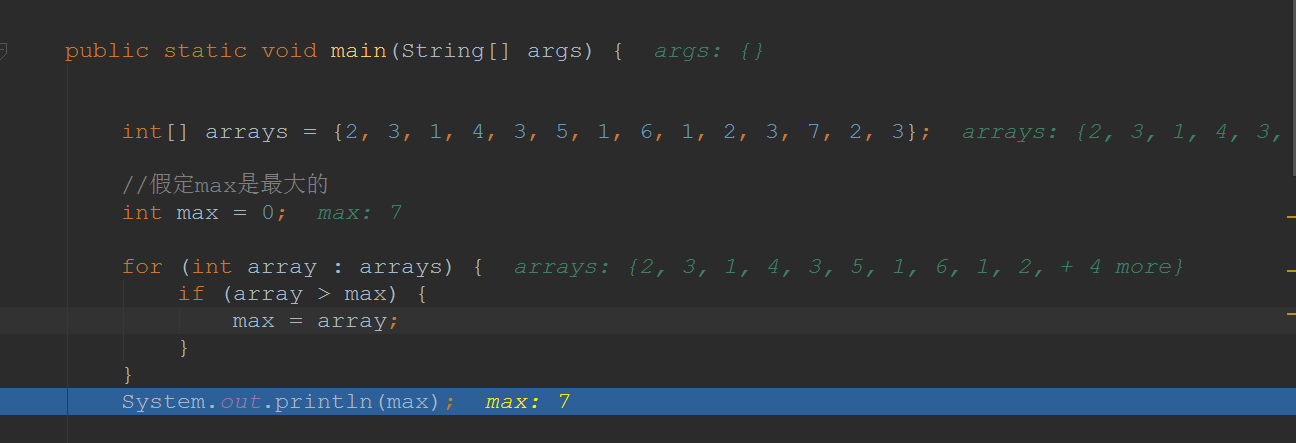//使用临时变量，让两个数互换
int temp;
temp = arrays;
arrays = arrays;
arrays = temp;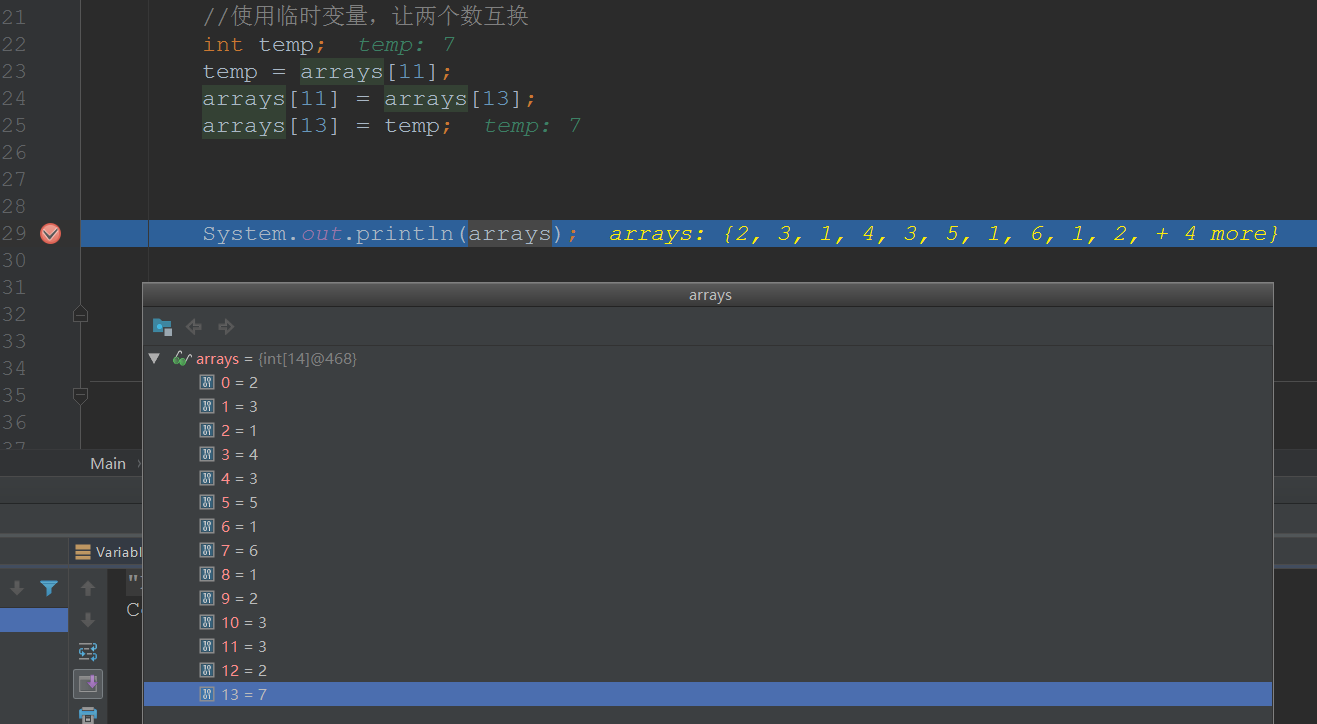## 二、第二趟排序


int max2 = 0;
for (int i = 0; i < (arrays.length - 1); i++) {
if (arrays[i] > max2) {
max2 = arrays[i];
}
}

System.out.println(max2);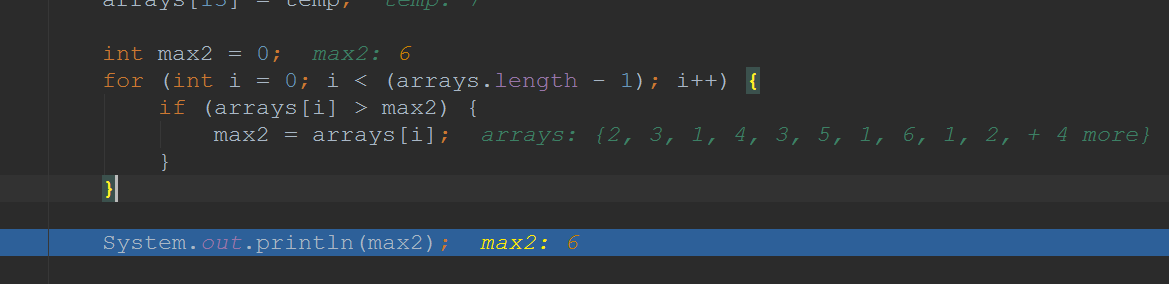temp = arrays;
arrays = arrays;
arrays = temp;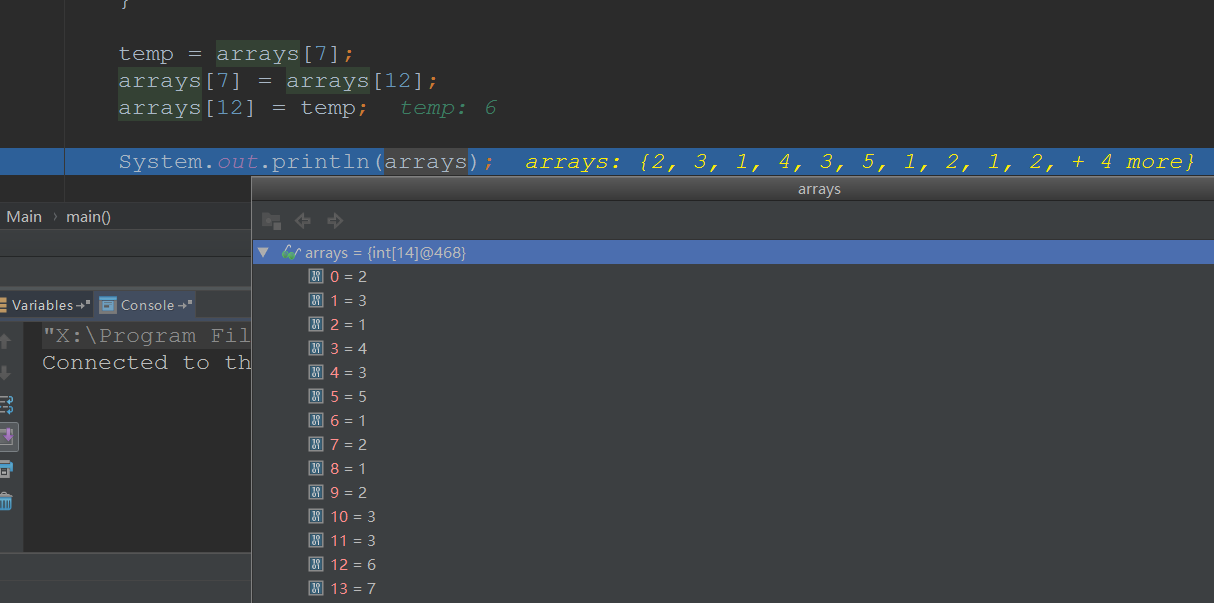## 三、代码简化

• 一个数组是需要n-1趟排序的(因为直到剩下一个元素时，才不需要找最大值)
• 每交换1次，再次找最大值时就将范围缩小1
• 查询当前趟数最大值实际上不用知道最大值是多少(上面我查出最大值，还要我手动数它的角标)，知道它的数组角标即可，交换也是根据角标来进行交换

....



//记录当前趟数的最大值的角标
int pos ;

//交换的变量
int temp;

//外层循环控制需要排序的趟数
for (int i = 0; i < arrays.length - 1; i++) {

//新的趟数、将角标重新赋值为0
pos = 0;

//内层循环控制遍历数组的个数并得到最大数的角标
for (int j = 0; j < arrays.length - i; j++) {

if (arrays[j] > arrays[pos]) {
pos = j;
}

}
//交换
temp = arrays[pos];
arrays[pos] = arrays[arrays.length - 1 - i];
arrays[arrays.length - 1 - i] = temp;

}

System.out.println("公众号Java3y" + arrays);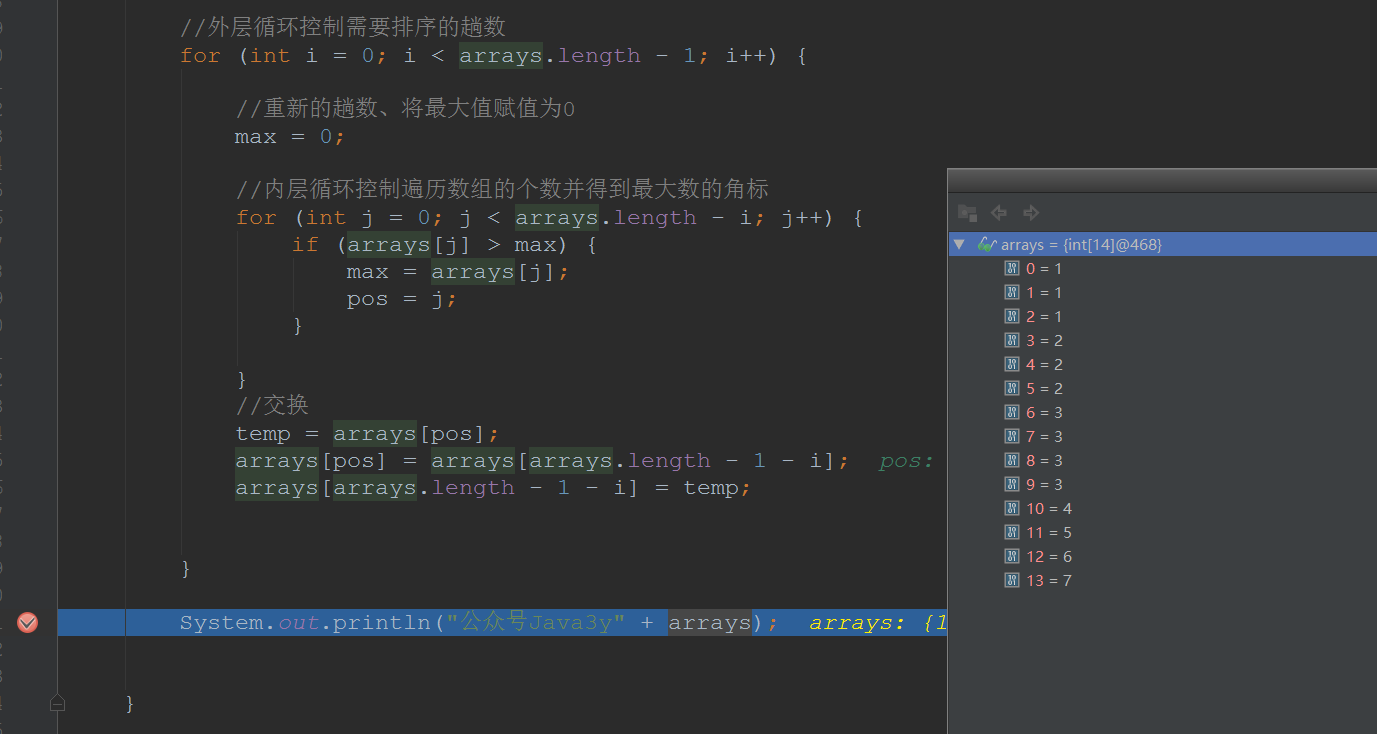## 五、扩展阅读

C语言实现

          int findMaxPos ( int arr[], int n)
{
int max = arr;
int pos = 0;
for (int i = 1; i < n; i++) {
if (arr[i] > max) {
max = arr[i];
pos = i;
}
}
return pos;
}

void selectionSort ( int arr[], int n)
{
while (n > 1)
{
int pos = findMaxPos(arr, n);
int temp = arr[pos];
arr[pos] = arr[n - 1];
arr[n - 1] = temp;
n--;//
}
}

int main ()
{
int arr[] = {5, 32, 7, 89, 2, 3, 4, 8, 9};
selectionSort(arr, 9);
for (int i = 0; i < 9; i++)
cout << arr[i] << endl;
}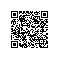使用钉钉扫一扫加入圈子
+ 订阅